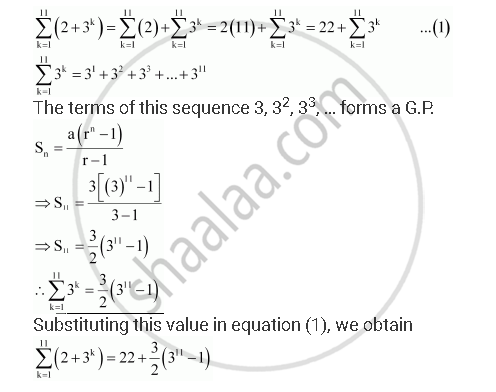CBSE (Arts) Class 11CBSE
Share
Notifications

View all notifications
Books Shortlist
Your shortlist is empty

# Geometric Progression Evaluate Sum_(K=1)^11 (2+3^K ) - CBSE (Arts) Class 11 - Mathematics

Login
Create free account

Forgot password?
ConceptGeometric Progression (G. P.)

#### Question

Evaluate sum_(k=1)^11 (2+3^k )

#### SolutionIs there an error in this question or solution?

#### APPEARS IN

NCERT Solution for Mathematics Textbook for Class 11 (2018 to Current)
Chapter 9: Sequences and Series
Q: 11 | Page no. 192
Solution Geometric Progression Evaluate Sum_(K=1)^11 (2+3^K ) Concept: Geometric Progression (G. P.).
S